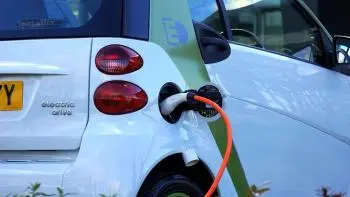# Dynamic Electricity: Electric Charges Movement and ExamplesDynamic electricity is a type of electricity that involves the movement of electrical charges through a conductor. This form of electricity is also known as electric current

Dynamic electricity production is based on the interaction between a magnetic field and an electric current moving through a conductor. This process is the basis for many electrical devices, such as electric motors, generators, and transformers, which convert mechanical energy into electrical energy and vice versa.

Electric charges can be positive or negative, but they are usually free (negatively charged) electrons.

## How Do Electric Charges Move?

Electric charges can move in a conducting material like metal from atom to atom. Electrical charges in a conductive material, such as electrons in a copper wire, move from one atom to another when an external electrical force is applied.

The electric potential drives the electrons of the atoms to jump to another atom further away. When the initial atom loses electrons, it becomes positively charged, which is why it attracts new electrons from previous atoms that have an excess of electrons and have been driven by the circuit's voltage. In this way, the electrons flow, generating an electric current.

## What Is the Difference Between Static and Dynamic Electricity?

On one side, static electricity refers to the buildup of electric charge on the surface of an insulator, typically caused by the transfer of electrons from one material to another through friction. The energy in a charged object remains static until it is discharged through a conductor or an arc.

Static electricity is responsible for many everyday phenomena, such as lightning, sparks, and shocks from touching a doorknob after walking on a carpet. In addition, different amperages can trigger the discharge of static electricity from a charged object.

On the other side dynamic electricity, on the other hand, refers to the flow of electric charge through a conductor, which is also known as an electric current. Dynamic electricity can be either direct current (DC) or alternating current (AC).

## Types of Electric Current

There are two main types of electrical current: direct current (DC) and alternating current (AC).

• Direct current (DC): Direct current flows in only one constant direction in the circuit. The electrical power source, such as a battery, provides a continuous electrical potential difference that drives current through the course.

• Alternating current (AC): Alternating current changes direction and magnitude at regular intervals, electrons do not travel long distances, but energy is transmitted through impulses. This current type is used in most electrical applications, including household, industrial, transportation, and power generation.

## Dynamic Electricity Examples

Here are some examples of dynamic electrical energy:

• Electricity produced by a hydropower station: In these power stations, the energy of moving water is converted into mechanical energy, which is then converted into an electrical current through an electrical generator.

• How an Electric Motor Works: An electric motor uses the flow of dynamic electricity to turn a rotor, which in turn produces mechanical work.

• Charging a battery: When a battery is charged, a flow of electrons is generated, transforming into chemical energy that can be converted back into electricity when required.

• The electrical energy generated by a wind turbine: When the blades of a wind turbine rotate, the wind's kinetic energy is converted into mechanical energy, which is converted into dynamic electricity through an electrical generator. This type of energy is known as wind power.

• The use of a transformer: A transformer uses dynamic electricity to create an electric field and change the voltage of an electric current, thus adapting it to the electrical circuit's needs.

Author:

Published: April 7, 2023
Last review: April 7, 2023